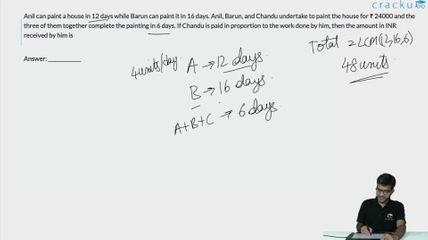Question 62

# Anil can paint a house in 12 days while Barun can paint it in 16 days. Anil, Barun, and Chandu undertake to paint the house for ₹ 24000 and the three of them together complete the painting in 6 days. If Chandu is paid in proportion to the work done by him, then the amount in INR received by him is

Solution

Now Anil Paints in 12 Days
Barun paints in 16 Days
Now together Arun , Barun and Chandu painted in 6 Days
Now let total work be W
Now each worked for 6 days
So Anil's work = 0.5W
Barun's work = $$\frac{6W}{16}=\frac{3W}{8}$$
Therefore Charu's work = $$\frac{W}{2}-\frac{3W}{8}=\ \frac{W}{8}$$
Therefore proportion of charu =$$\frac{24000}{8}=\ 3,000$$

### View Video Solution